# Chapter 13 Statistics and Probability NCERT Exemplar Solutions Exercise 13.3 Class 10 Maths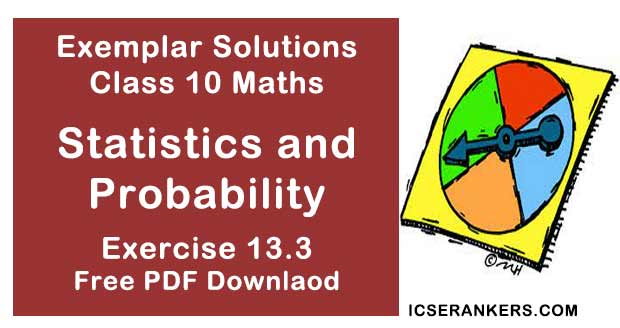Chapter Name NCERT Maths Exemplar Solutions for Chapter 13 Statistics and Probability Exercise 13.3 Book Name NCERT Exemplar for Class 10 Maths Other Exercises Exercise 13.1Exercise 13.2Exercise 13.4 Related Study NCERT Solutions for Class 10 Maths

### Exercise 13.3 Solutions

1. Find the mean of the distribution

 Class 1 – 3 3 – 5 5 – 7 7 – 10 Frequency 9 22 27 17

Solution

We first, find the class mark xi, of each class and then proceed as follows.
 Class Class marks(xi) Frequency(fi) fi xi 1 – 3 2 9 18 3 – 5 4 22 88 5 – 7 6 27 162 7 – 10 8.5 17 144.5 Σfi = 75 Σfi xi = 412.5

Therefore, mean (x) = (Σfi xi)/(Σfi) = 412.5/75 = 5.5
Hence, mean of the given distribution is  5.5.

2. Calculate the mean of the scores of 20 students in a mathematics test
 Marks 10 – 20 20 – 30 30 – 40 40 – 50 50 – 60 Number of students 2 4 7 6 1
Solution
We first, find the class mark of each class and then proceed as follows
 Marks Class marks(xi) Frequency(fi) fi xi 10 – 20 15 2 30 20 – 30 25 4 100 30 – 40 35 7 245 40 – 50 45 6 270 50 – 60 55 1 55 Σfi = 20 Σfi xi = 700

Therefore, mean (x) = (Σfi xi)/(Σfi) = 700/20 = 35
Hence, the mean of scores of  20 students in mathematics test is  35.

3. Calculate the mean of the following data

 Class 4 – 7 8 – 11 12 – 15 16 – 19 Frequency 5 4 9 10
Solution
Since, given data is not continuous, so we subtract 0.5 from the lower limit and add 0.5 in
the upper limit of each class.
Now, we first find the class mark xi, of each class and then proceed as follows
 Class Class marks(xi) Frequency(fi) fi xi 3.5 - 7.5 5.5 5 27.5 7.5 – 11.5 9.5 4 38 11.5 – 15.5 13.5 9 121.5 15.5 – 19.5 17.5 10 175 Σfi = 28 Σfi xi = 362

Therefore, mean (x) = (Σfi xi)/(Σfi)= 362/28 = 12.93
Hence, mean of the given data is 12.93.

4. The following table gives the number of pages written by Sarika for completing her own book for 30 days.
 Number of pages written per day 16 – 18 19 – 21 22 – 24 25 – 27 28 – 30 Number of days 1 3 4 9 13
Find the mean number of pages written per day.
Solution
Since,
 Class - marks Mid - value(xi) Number of days(fi) fi xi 15.5 – 18.5 17 1 17 18.5 – 21.5 20 3 60 21.5 – 24.5 23 4 92 24.5 – 27.5 26 9 234 27.5 – 30.5 29 13 377 Total Σfi = 30 Σfi xi = 780
Since, given data is not continuous, so we subtract 0.5 from the lower limit and add 0.5 in the upper limit of each class.
∴  mean (x) = (Σfi xi)/(Σfi) = 780/30 = 26
Hence, the mean of pages written per day is 26.

5. The daily income of a sample of 50 employees are tabulated as follows.
 Income (in ₹) 1 – 200 201 – 400 400 – 600 601 – 800 Number of employees 14 15 14 7
Find the mean daily income employees.
Solution
Since, given data is not continuous, so we subtract 0.5 from the lower limit and add 0.5 in
the upper limit of each class.
Now we first, find the class mark xi, of each class and then proceed as follows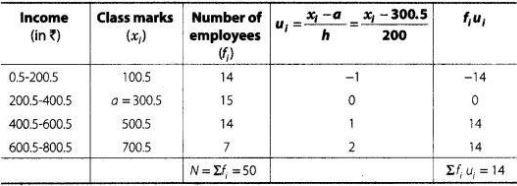∴ Assumed mean,  a = 300.5
Class width, h = 200
and total observations, N = 50
By step deviation method,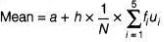= 300.5 + 200 × (1/50) × 14
= 300.5 + 56 = 356.5

6. An aircraft has 120 passenger seats. The number of seats occupied during 100 flights is given in the following table.
 Number of seats 100 – 104 104 – 108 108 – 112 112 – 116 116 – 120 Freqency 15 20 32 18 15

Determine the mean number of seats occupied over the flights.

Solution

We first, find the class mark xi, of each class and then proceed as follows.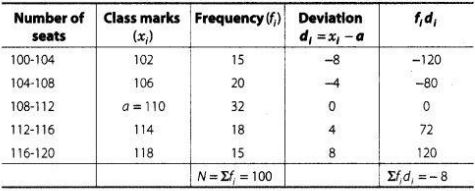∴ Assumed mean, a = 110,
Class width, h = 4
and total observation, N = 100
By assumed mean method,
Mean ( x ) = a +  (Σfi di )/(Σfi )
= 110 + (-8/100) = 110 - 0.08 = 109.92

7. The weights (in kg) of 50 wrestlers are recorded in the following table. Find the mean weight of the wrestlers.
 Weight (in kg) 100 – 110 110 – 120 120 – 130 130 – 140 140 – 150 Number of wrestlers 4 14 21 8 3
Solution
We first the class mark of each class and then proceed as follows :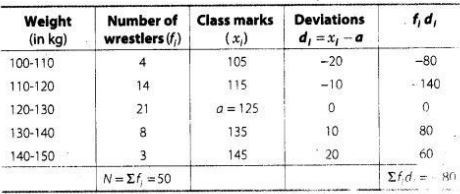∴ Assumed mean (a) = 125
Class width (h) = 10 and total observation (N) = 50
By assumed mean method,
Mean (x) = a +  (Σfi di)/(Σfi)
= 125 + (-80)/50
= 125 - 16 = 123.4 kg

8. The mileage (km per litre) of 50 cars of the same model was tested by a manufacturer and
details are tabulated as given below
 Mileage(kmL-1 ) 10 – 12 12 – 14 14 – 16 16 – 18 Number of cars 7 12 18 13
Find the mean mileage.
The manufacturer claimed that the mileage of the model was 16 kmL -1.
Do you agree with this claim?
SolutionHere, Σfi = 50
and Σfi xi = 724
∴ Mean (x) = (Σfi xi)/Σfi
= 724/50 = 14.48
Hence, mean mileage is 14.48 kmL-1 .
No, the manufacturer is claiming mileage 1.52 kmh-1 more than average mileage.

9. The following is the distribution of weights (in kg) of 40 persons.
 Weight (in kg) 40 – 45 45 – 50 50 – 55 55 – 60 60 – 65 65 – 70 70 – 75 75 – 80 Number of persons 4 4 13 5 6 5 2 1
Construct a cumulative frequency distribution (of the less than type) table for the data above.
Solution
The cumulative distribution (less than type) table is shown below
 Weight (in kg) Cumulative frequency Less than 45 4 Less than 50 4 + 4 = 8 Less than 55 8 + 13 = 21 Less than 60 21 +5 = 26 Less than 65 26 + 6 = 32 Less than 70 32 + 5 = 37 Less than 75 37 + 2 = 39 Less than 80 39 + 1 = 40

10. The following table shows the cumulative frequency distribution of marks of 800 students in an examination.

 Marks Number of students Below 10 10 Below 20 50 Below 30 130 Below 40 270 Below 50 440 Below 60 570 Below 70 670 Below 80 740 Below 90 780 Below 100 800
Construct a frequency distribution table for the data above.
Solution
Here, we observe that 10 students have scored marks below 10 i.e., it lies between class interval 0-10. Similarly, 50 students have scored marks below 20. So, 50 -10 = 40 students lies in the interval 10-20 and so on. The table of a frequency distribution for the given data is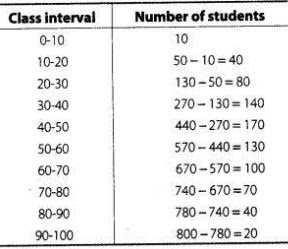11. From the frequency distribution table from the following data.
 Marks (Out of 90) Number of candidates More than or equal to 80 4 More than or equal to 70 6 More than or equal to 60 11 More than or equal to 50 17 More than or equal to 40 23 More than or equal to 30 27 More than or equal to 20 30 More than or equal to 10 32 More than or equal to 0 34
Solution
Here, we observe that, all 34 students have scored marks more than or equal to 0. Since, 32 students have scored marks more than or equal to 10. So, 34- 32 =2 students lies in the interval 0-10 and so on.
Now, we construct the frequency distribution table.
 Class interval Number of candidates 0 – 10 34 – 32 = 2 10 – 20 32 – 30 = 2 20 – 30 30 – 27 = 3 30 – 40 27 – 23 = 4 40 – 50 23 – 17 = 6 50 – 60 17 – 11 = 6 60 – 70 11 – 6 = 5 70 – 80 6 – 4 = 2 80 – 90 4

12. Find the unknown entries o, b, c, d, e and f in the following distribution of heights of students in a class

 Height (in cm) Frequency Cumulative frequency 150 – 155 12 A 155 – 160 b 25 160 – 165 10 C 165 – 170 d 43 170 – 175 e 48 175 – 180 2 f Total 50
Solution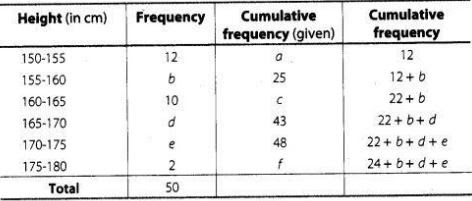On comparing last two tables, we get
a = 12
∴ 12 + b = 25
⇒ b = 25 - 12 = 13
22 + b = c
⇒ c = 22 + 13 = 35
22 + b + d = 43
⇒ 22 + 13 + d = 43
⇒ d = 43 - 35 = 8
and 22 + b + d + e = 48
⇒ 22 + 13 + 8 + e = 48
⇒ e = 48 - 43 = 5
and 24 + b + d + e = f
⇒ 24 + 13 + 8 + 5 = f
∴  f = 50

13. The following are the ages of 300 patients getting medical treatment in a hospital on a particular day
 Age (in year) 10 – 20 20 – 30 30 – 40 40 – 50 50 – 60 60 – 70 Number of patients 60 42 55 70 53 20
Form
(i) less than type cumulative frequency distribution.
(ii) More than type cumulative frequency distribution.
Solution
(i) We observe that the number of patients which take medical treatment in a hospital on a particular day less than 10 is O’. Similarly, less than 20 include the number of patients which take medical treatment from 0-10 as well as the number of patients which take medical treatment from 10-20.
So, the total number of patients less than 20 is 0 + 60 = 60, we say that the cumulative frequency of the class 10-20 is 60. Similarly, for other class.

(ii) Also, we observe that all 300 patients which take medical treatment more than or equal to 10. Since, there are 60 patients which take medical treatment in the interval 10-20, this means that there are 300 –60 = 240 patients which take medical treatment more than or equal to 20. Continuing in the same manner.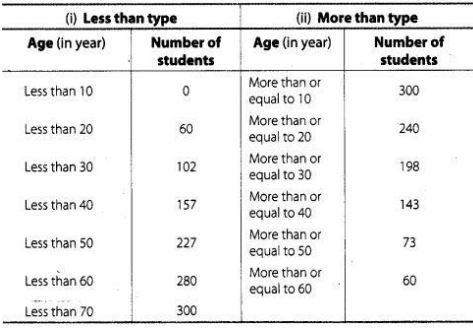14. Given below is a cumulative frequency distribution showing the marks secured by 50 students of a class
 Marks Below 20 Below 40 Below 60 Below 80 Below 100 Number of students 17 22 29 37 50

Form the frequency distribution table for the data.

Solution

Here, we observe that, 17 students have scored marks below 20 i.e., it lies between class interval 0-20 and 22 students have scored marks below 40, so 22 -17 = 5 students lies in the class interval 20-40 continuing in the same manner, we get the complete frequency distribution table for given data.
 Marks Number of students 0 – 20 17 20 – 40 22 – 17 = 5 40 – 60 29 – 22 = 7 60 – 80 37 – 29 = 8 80 – 100 50 – 37 = 13

15. Weekly income of 600 families is tabulated below
 Weekly Income(in ₹) Number of families 0 – 1000 250 1000 – 2000 190 2000 – 3000 100 3000 – 4000 40 4000 – 5000 15 5000 – 6000 5 Total 600
Compute the median income
Solution
First we construct a cumulative frequency table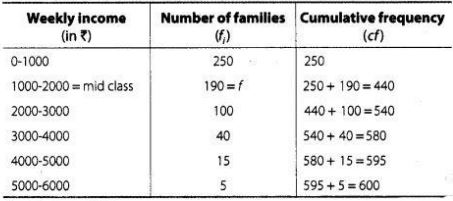It is given that, n = 600
∴ n/2 = 600/2 = 300
Since, cumulative frequency 440 lies in the interval 1000 - 2000.
Here, (lower median class)l = 1000,
f = 190, cf = 250, (class width) h = 1000
and (total observation ) n = 600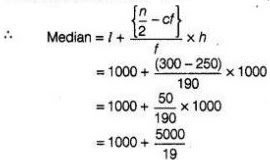= 1000 + 263.15 = 1263.15
Hence, the median income is ₹ 1263.15.

16. The maximum bowling speeds, in km per hour, of 33 players at a cricket coaching centre are given as follows
 Speed (in km/h) 85 – 100 100 – 115 115 – 130 130 – 145 Number of players 11 9 8 5
Calculate the median bowling speed.
Solution
First we construct the cumulative frequency table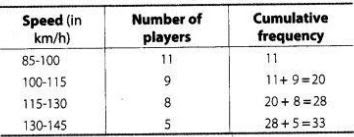It is given that, n = 33
∴ n/2 = 33/2 = 16.5
So, the median class is  100 - 115,
where, lower limit (l) = 100,
frequency (f) = 9,
cumulative frequency (cf) = 11
and class width (h) = 15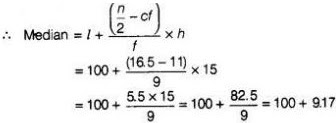= 109.17
Hence, the median bowling speed is 109.17 km/h.

17. The monthly income of  100 families are given as below
 Income (in ₹) Number of families 0 – 5000 8 5000 – 10000 26 10000 – 15000 41 15000 – 20000 16 20000 – 25000 3 25000 – 30000 3 30000 – 35000 2 35000 – 40000 1

Calculate the model income.

Solution
In a given data, the highest frequency is 41, which lies in the interval 10000-15000.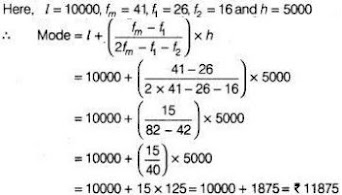Hence, the modal income is ₹ 11875.

18. The weight of coffee in  70 packets are shown in the following table
 Weight (in g) Number of packets 200 – 201 12 201 – 202 26 202 – 203 20 203 – 204 9 204 – 205 2 205 – 206 1

Determine the model weight.

Solution

In the given data, the highest frequency is 26, which lies in the interval 201 - 202.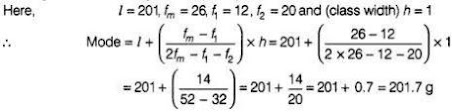Hence, the modal weight is 201.7 g.

19. Two dice are thrown at the same time. Find the probability of getting
(i) same number on both dice.
(ii) different number on both dice.
Solution
Two dice are thrown at the same time. [given]
So, total number of possible outcomes = 36
(i) We have, same number on both dice.
So, possible outcomes are (1,1), (2, 2), (3, 3), (4, 4), (5, 5) and (6, 6).
∴ Number of possible outcomes = 6
Now, required probability = 6/36 = 1/6

(ii) We have, different number on both dice.
So, number of possible outcomes
= 36 - Number of possible outcomes for same number on both dice
= 36 - 6 = 30
∴ Required probability = 30-36 = 5/ 6

20. Two dice are thrown simultaneously. What is the probability that the sum of the numbers appearing on the dice is
(i) 7 ?
(ii) a prime number ?
(iii) 1 ?
Solution
Two dice are thrown simultaneously. [given]
So, total number of possible outcomes = 36

(i) Sum of the numbers appearing on the dice is 7.
So, the possible ways are (1, 6), (2, 5), (3, 4), (4, 3) , (5, 2) and  (6, 1 )
Number of possible ways = 6
∴ Required probability = 6/36 = 1/6

(ii) Sum of the numbers appearing on the dice is a prime number i.e. 2, 3, 5, 7 and 11.
So, the possible ways are (1, 1), (1, 2), (2, 1), (1, 4), (2, 3), (3, 2), (4, 1), (1, 6), (2, 5), (3, 4), (4, 3), (5, 2), (6, 1), (5, 6) and (6, 5) .
Number of possible ways = 15
∴ Required probability = 15/36 = 5/12

(iii) Sum of the numbers appearing on the dice is 1.
It is not possible, so its probability is zero.

21. Two dice are thrown together. Find the probability that the product of the numbers on the top of the dice is
(i) 6
(ii) 12
(iii) 7
Solution
Number of total outcomes = 36

(i) When product of the numbers on the top of the dice is  6.
So, the possible ways are (1, 6), (2, 3), (3, 2) and (6, 1).
Number of possible ways  = 4
∴ Required probability = 4/36 = 1/9

(ii) When product of the numbers on the top of the dice is 12.
So, the possible ways are (2, 6), (3, 4), (4, 3) and (6, 2).
Number of possible ways = 4
∴ Required probability = 4/36 = 1/9

(iii) Product of the numbers on the top of the dice cannot be 7. So, its probability is zero.

22. Two dice are thrown at the same time and the product of numbers appearing on them is noted. Find the probability that the product is less than 9.
Solution
Number of total outcomes = 36
When product of numbers appearing on them is less than 9, then possible ways are (1,6),
(1,5) (1,4), (1,3), (1,2), (1,1), (2, 2), (2, 3), (2, 4), (3, 2), (4, 2), (4,1), (3,1), (5,1), (6,1) and (2,1).
Number of possible ways = 16
Required probability = 16/36 = 4/9

23. Two dice are numbered 1, 2, 3, 4, 5, 6 and 1, 1, 2, 2, 3, 3, respectively. They are thrown and the sum of the numbers on them is noted. Find the probability of getting each sum from 2 to 9, separately.
Solution
Number of total outcomes = 36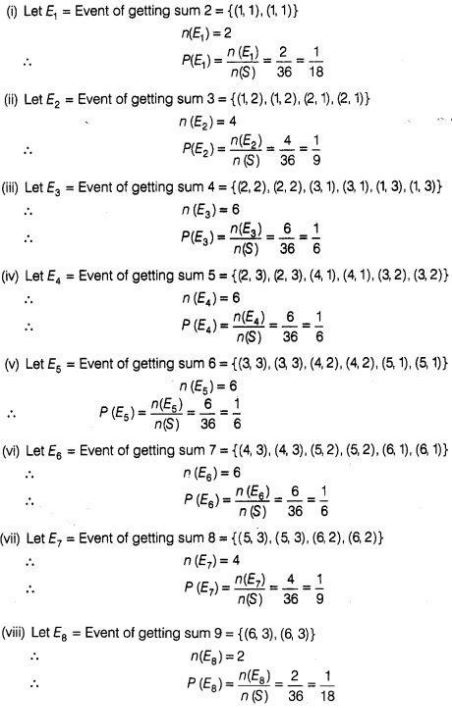24. A coin is tossed two times. Find the probability of getting at most one head.
Solution
The possible outcomes, if a coin is tossed 2 times is
S = {(HH), (TT), (HT), (TH)}
∴ n(S) = 4
Let E = Event of getting at most one head
= {(TT), (HT), (TH)}
∴ n(E) = 3
Hence, required probability = n(E)/n(S) = 3/4

25. A coin is tossed 3 times. List the possible outcomes. Find the probability of getting
Solution
The possible outcomes if a coin is tossed 3 times is
S = {(HHH), (TTT), (HTT), (THT), (TEH), (THH), (HTH), (HHT)}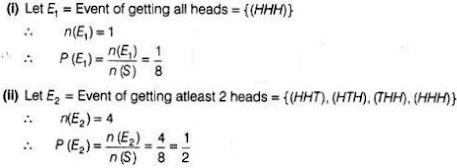26. Two dice are thrown at the same time. Determine the probability that the difference of the
numbers on the two dice is 2.
Solution
The total number of sample space in two dice, n(S) = 6 × 6 = 36
Let,   E = Event of getting the numbers whose difference is 2
= {(1, 3), (2, 4), (3, 5), (4, 6), (3, 1), (4, 2), (5, 3), (6, 4)}
∴ n(E) = 8
∴ P(E)  = n(E)/n(S) = 8/36 = 2/9

27. A bag contains 10 red, 5 blue and 7 green balls. A ball is drawn at random. Find the probability of this ball being a
(i) red ball
(ii) green ball
(iii) not a blue ball
Solution
if a ball is drawn out of 22 balls (5 blue + 7 green + 10 red), then the total number of outcomes are
n(S) = 22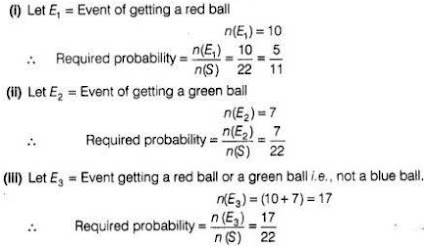28. The king, queen and jack of clubs are removed from a deck of 52 playing cards and then well shuffled. Now, one card is drawn at fandom from the remaining cards. Determine the probability that the card is
(i) a heart
(ii) a king
Solution
If we remove one king, one queen and one jack of clubs from 52 cards, then the remaining cards left, n(S) = 49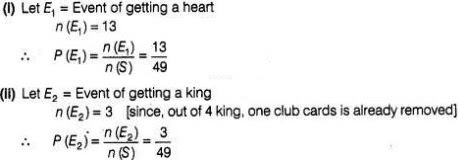29. Refer to Q.28. What is the probability that the card is
(i) a club
(ii) 10 of hearts
Solution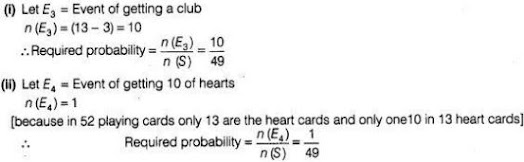30. All the jacks, queens and kings are removed from a deck of 52 playing cards. The remaining cards are well shuffled and then one card is drawn at random. Giving ace a value 1 similar value for other cards, find the probability that the card has a value.
(i) 7
(ii) greater than 7
(iii) Less than 7
Solution
In out of 52 playing cards, 4 jacks, 4 queens and 4 kings are removed, then the remaining
cards are left, n(S) = 52 – 3 × 4 = 40.

(i) Let E1 = Event of getting a card whose value is 7
E = Card value 7 may be of a spade, a  diamond, a club or a heart
∴ n(E1) = 4
∴ P(E1) = n(E1)/n(S) = 4/40 = 1/10

(ii) Let E2 = Event of getting a card whose value is greater than 7
= Event of getting a card whose value is 8, 9 or 10
∴ n(E2) = 3 × 4 = 12
P(E2 ) = n(E2)/n(S) = 12/40 = 3/10

(iii) Let E3 = Event of getting a card whose value is less than 7
= Event of getting a card whose value is 1, 2, 3, 4, 5 or  6
∴   n(E3) = 6 × 4 = 24
∴  P(E3) = n(E3)/n(S) = 24/40 = 3/5

31. An integer is chosen between 0 and 100. What is the probability that it is
(i) divisible by 7?
(ii) not divisible by 7?
Solution
The number of integers between 0 and  100 is
n(S)= 99

(i) Let E1 = Event of choosing an integer which is divisible by 7
= Event of choosing an integer which is multiple of 7
= {7, 14, 21, 28, 35, 42, 49, 56,63, 70, 77, 84, 91, 98}
∴  n(E1) = 14
∴ P(E1) = n(E1)/n(S) = 14/99

(ii) Let E2 = Event of choosing an integer which is not divisible by 7
∴ n(E2) = n(S) - n(E1) = 99 - 14 = 85
P(E2) = n(E2)/n(S) = 85/99

32. Cards with numbers 2 to 101 are placed in a box. A card is selected at random. Find the probability that the card has
(i) an even number
(ii) a square number
Solution
Total number of out comes with numbers  2 to 101, n(S) = 100
(i) Let E1 = Event of selecting a card which is an even number = {2, 4, 6, ....100}
[in an AP, l = a + (n-1)d, here l = 100, a = 2 and d = 2
⇒ 100 = 2 + (n - 1)2
⇒ (n - 1) = 49 ⇒ n = 50]
∴ n(E1 ) = 50
∴ Required probability = n(E1 )/n(S) = 50/100 = 1/2

(ii) Let E2 = Event of selecting a card which is a square number
= {4, 9, 16, 25, 36, 49, 64, 81, 100}
= {(2)2 ,(3)2 , (4)2 , (5)2 , (6)2 , (7)2 , (8)2 , (9)2 , (10)2 }
∴ n(E2 ) = 9
Hence, required probability = n(E2 )/n(S) = 9/100

33. A letter of english alphabets is chosen at random. Determine the probability that the letter is a consonant.
Solution
We know that, in english alphabets, there are (5 vowels + 21 consonants)=26 letters. So, total number of outcomes in english alphabets
are, n(S) = 26
Let  E = Event of choosing a english alphabet, which is a consonant
= {b, c, d, f, g, h, j, k, l, m, n, p, q, r, s, t, v, w, x, y, z}
∴ n(E) = 21
Hence, required probability = n(E)/n(S) = 21/26

34. There are 1000 sealed envelopes in a box, 10 of them contain a cash prize of ₹ 100 each, 100 of them contain a cash prize of ₹ 50 each and 200 of them contain a cash prize of ₹ 10 each and rest do not contain any cash prize. If they are well shuffled and an envelope is picked up out, what is the probability that it contains no cash prize?
Solution
Total number of sealed envelopes in a box, n (S) = 1000
Number of envelopes containing cash prize = 10 + 100 + 200 = 310
Number of envelopes containing no cash prize,
n(E) = 1000 - 310 = 690
∴ P(E) = n(E)/n(S) = 690/1000 = 69/100 = 0.69

35. Box A contains 25 slips of which 19 are marked ₹ 1 and other are marked ₹ 5 each. Box B contains 50 slips of which 45 are marked ₹ 1 each and others are marked ₹ 13 each. Slips of both boxes are poured into a third box and resuffled. A slip is drawn at random. What is the probability that it is marked other than ₹ 1?
Solution
Total number of slips in a box, n(S) = 25 + 50 = 75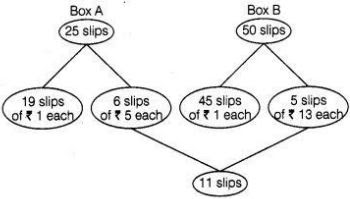From the chart it is clear that, there are 11 slips which are marked other than ₹ 1.
∴ Required probability  = (Number of slips other than ₹ 1)/(Total number of slips) = 11/75

36. A carton of 24 bulbs contain 6 defective bulbs. One bulb is drawn at random. What is the probability that the bulb is not defective? If the bulb selected is defective and it is not replaced and a second bulb is selected at random from the rest, what is the probability that the second bulb is defective?
Solution
∴ Total number of bulbs, n(S) = 24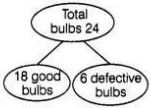Let E1 = Event of selecting not defective bulb = Event of selecting good bulbs
n(E1) = 18
∴ P(E1) = n(E1)/n(S) = 18/24 = 3/4
Suppose, the selected bulb is defective and not replaced, then total number of bulbs  remains in a carton, n(S) = 23.
In them, 18 are good bulbs and 5 are defective bulbs.
∴ P (selecting second defective bulb) = 5/23

37.  A child’s game has 8 triangles of which 3 are blue and rest are red, and 10 squares of which 6 are blue and rest are red. One piece is lost at random. Find the probability that it is a
(i) triangle
(ii) square
(iii) square of blue colour
(iv) triangle of red
Solution
Total number of figures
n(S) = 8 triangles + 10 squares = 18
(i) P (lost piece is a triangle ) = 8/18 = 4/9
(ii) P (lost piece is a square) = 10/18 = 5/9
(iii) P (square of blue colour) = 6/18 = 1/3
(iv) P(triangle of red colour) = 5/18

38. In a game, the entry fee is of ₹ 5. The game consists of a tossing a coin 3 times. If one or two heads show, Sweta gets her entry fee back. If she throws 3 heads, she receives double the entry fees. Otherwise she will lose. For tossing a coin three times, find the probability that she
(i) loses the entry fee.
(ii) gets double entry fee.
(iii) just gets her entry fee.
Solution
Total possible outcomes of tossing a coin 3 times,
S = {(HHH), (TTT), (HTT), (THT), (TTH), (THH), (HTH), (HHT)}
∴ n (S) = 8
(i) Let E1 = Event that Sweta losses the entry fee
= She tosses tail on three times
n(E1) = {(TTT)}
P(E1) = n(E1)/n(S) = 1/8

(iii) Let E3 = Event that Sweta gets her entry fee back
= Sweta gets heads one or two times
= (HTT), (THT), (TTH), (HHT) , (HTH), (THH)}
∴  n(E3) = 6
∴ P(E3) = n(E3)/n(S) = 6/8 = 3/4

39. A die has its six faces marked 0, 1, 1, 1, 6, 6. Two such dice are thrown together and the total
score is recorded.
(i) How many different scores are possible?
(ii) What is the probability of getting a total of 7 ?
Solution
Given, a die has its six faces marked {0,1,1,1,6, 6}
Total sample space, n(S) = 62 = 36

(i) The different score which are possible are 6 scores e., 0,1,2,6,7 and12.

(ii) Let E = Event of getting a sum 7
= {(1, 6) , (1, 6), (1, 6), (1, 6), (1, 6), (1, 6), (6, 1), (6, 1), (6, 1), (6, 1), (6, 1), (6, 1)
∴ n(E) = 12
∴ P(E) = n(E)/n(S) = 12/36 = 1/3

40. A lot consists of 48 mobile phones of which 42 are good, 3 have only minor defects and 3 have major defects. Varnika will buy a phone, if it is good but the trader will only buy a mobile, if it has no major defect. One phone is selected at random from the lot. What is the probability that it is
(i) acceptable to Varnika?
Solution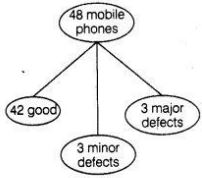Given, total number of mobile phones
n(S) = 48
(i) Let E1 = Event that Varnika will buy a mobile phone
= Varnika buy only, if it is good mobile
∴ n(E1)= 42
∴ P(E1) = n(E1 )/n(S) = 42/48 = 7/8

(ii) Let E2 = Event that trader will buy only when it has no major defects
∴ n(E2) = 45
∴ P(E2) = n(E2)/n(S) = 45/48 = 15/16

41. A bag contains 24 balls of which x are red, 2x are white and 3x am are. A ball is selected at random. What is the probability that it
(i) not red?
(ii) white
Solution
Given that, A bag contains total number of balls = 24 A bag contains number of red bails = 24
A bag contains number of white balls = 2x and a bag contains number of blue balls = x
By condition, x + 2x + 3x = 24
⇒ 6x = 24
∴ x = 4
∴ Number of red balls = x = 4
Number of white balls = 2x = 2 ×4 = 8
and number of blue balls = 3x = 3 × 4 = 12
So, total number of outcomes for a ball is selected at random in a bag contains 24 balls.
⇒ n(S) = 24

(i) Let E1 = Event of selecting a ball which is not red i.e., can be white or blue,
∴ n(E1) = Number of white balls + Number of blue balls
⇒ n(E1) = 8 + 12 = 20
∴ Required probability = n(E1)/n(S) = 20/24 = 5/6

(ii) Let E2 = Event of selecting a ball which is white
∴ n(E2) = Number of white balls = 8
So, required probability = n(E2)/n(S) = 8/24 = 1/3

42. At a fete, cards bearing numbers 1 to 1000, one number on one card, are put in a box. Each
player selects one card at random and that card is not replaced. If the selected card has a perfect square greater than 500, the player wins a prize. What is the probability that
(i) the first player wins a prize?
(ii) the second player wins a prize, if the first has won?
Solution
Given that,, at a fete, cards bearing numbers 1 to 1000 one number on one card, are put in a
box. Each player selects one card at random and that card is not replaced so, the total number of outcomes are n(S) = 1000
If the selected card has a perfect square greater than 500, then player wins a prize.

(i) Let E1 = Event first player wins a prize = Player select a card  which is a perfect square greater than 500
= { 529, 576, 625, 676, 729, 784, 841, 900, 961}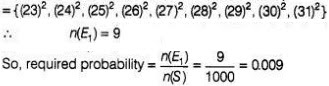(ii) First, has won i.e., one card is already selected, greater than 500, has a perfect square. Since, repeatition is not allowed. So, one card is removed out of 1000 cards. So, number of remaining cards is  999.
∴ Total number of remaining outcomes, n(S') = 999
Let E2 = Event the second player wins a prize, if the first has won.
= Remaining cards has a perfect square greater than 500 are 8.
∴ n(E2) = 9 - 1 = 8
So, required probability  = n(E2)/n(S') = 8/999